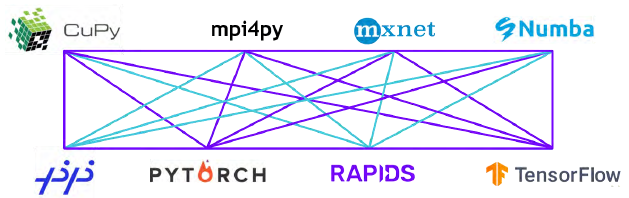# What is Regression?

Classification and regression are two groups of supervised machine learning algorithms problems. Supervised machine learning uses algorithms to train a model to find patterns in a dataset with labels and features. Classification predicts which category an item belongs to based on labeled examples of known items. Regression estimates the relationship between a target outcome label and one or more feature variables to predict a continuous numeric value. Many supervised learning algorithms, such as decision trees, can be used for classification or regression.

Statistical regression analysis mathematically determines the relationship between a dependent variable and one or more independent variables. There are numerous types of regression algorithms. Linear regression is an algorithm used for regression to predict a numeric value, for example the price of a house. Logistic regression is an algorithm used for classification to predict the probability that an item belongs to a class, for example the probability that an email is spam.

## What is Linear Regression?

Linear regression fits a linear model through a set of data points to estimate the relationship between a target outcome label and one or more feature variables in order to predict a numeric value. The output y (label) can be estimated as a straight line that can be visualized as:

y = intercept +  ci * xi + Error

Where xi are the input variables (features), and the parameters ci, intercept, and Error are the regression coefficients, the constant offset, and the error respectively. The coefficients ci can be interpreted as the increase in a dependent variable (y label) for a unit increase in the respective independent variable (x feature). In the simple example below, linear regression is used to estimate the house price (the y label) based on the house size (the x feature).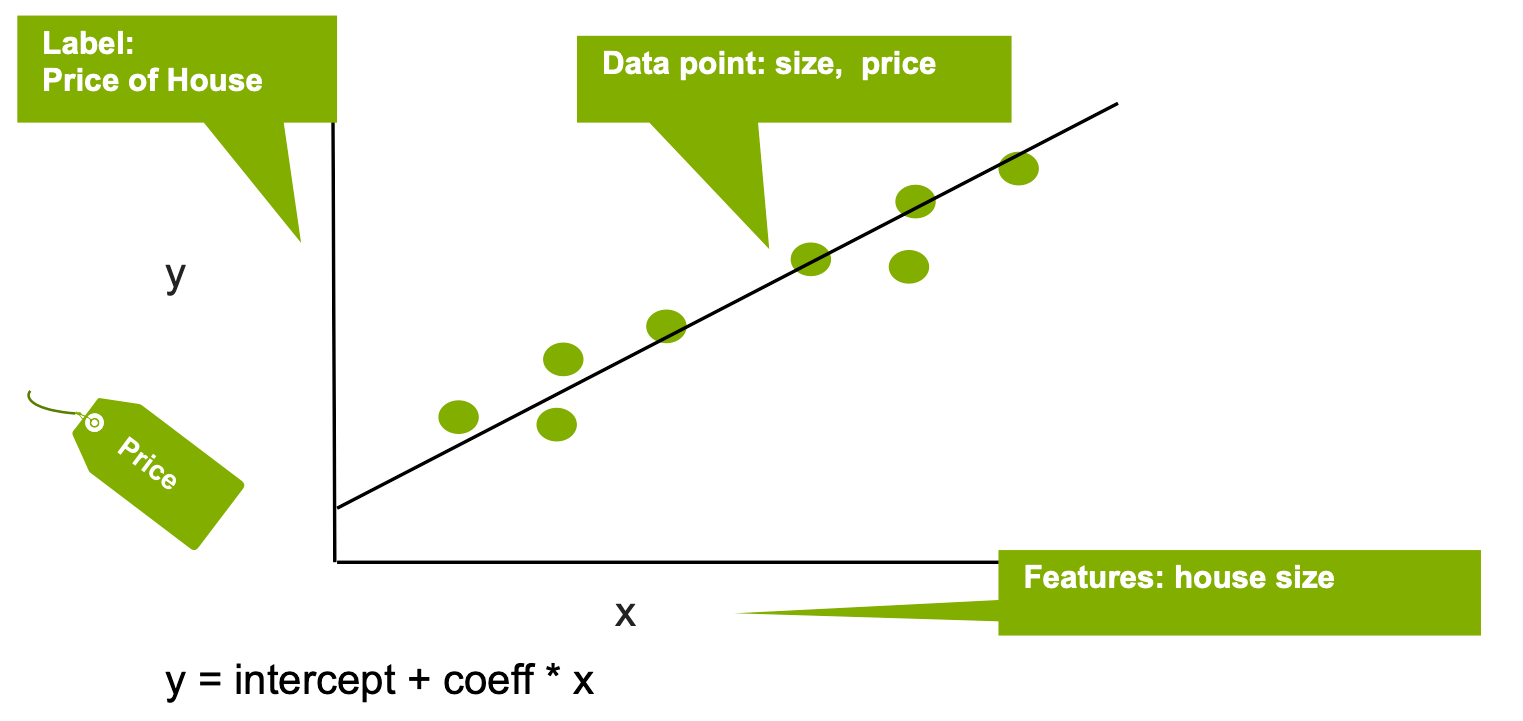The distance between the x and y points and the line determines the strength of the connection between the independent and dependent variables. The slope of the line is often determined using the least squares method in which the sum of the squares of the offsets of points on the curve is minimized.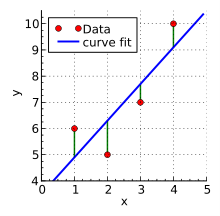Source: Wikipedia

There are two basic types of linear regression—simple linear regression and multiple linear regression. In simple linear regression, one independent variable is used to explain or predict the outcome of a single dependent variable. Multiple linear regression does the same thing using two or more independent variables.

Regression is often used for predicting outcomes. For example, regression might be to find the correlation between tooth brushing and tooth decay. The x-axis is the frequency of cavities in a given population and the y-axis is the frequency with which people in that population brush their teeth. Each person is identified by a dot on the chart representing their weekly brushing frequency and the number of cavities they have. In a real-world case, there would be dots all over the chart, as even some people who brush frequently get cavities while some people who don’t brush very often are spared from tooth decay. However, given what is known about tooth decay, a line that comes closest to touching all the points on the chart will probably slope down and to the right.

One of the most useful applications of regression analysis is the weather. When a strong correlation can be established between variables–such as ocean temperatures in the southeastern Atlantic and the incidence of hurricanes–a formula can be created to predict future events based upon changes in the independent variables.

Regression analysis can also be useful in financial scenarios such as predicting the future value of an investment account based upon historical interest rates. While interest rates vary from month to month, over the long term, certain patterns emerge that can be used to forecast the growth of and investment with a reasonable degree of accuracy.

The technique is also useful in determining correlations between factors whose relationship is not intuitively obvious. However, it’s important to remember that correlation and causation are two different things. Confusing them can lead to dangerous misassumptions. For example, ice cream sales and the frequency of drowning deaths are correlated due to a third factor–summer–but there’s no reason to believe that eating ice cream has anything to do with drowning.

This is where multiple linear regression is useful. It examines several independent variables to predict the outcome of a single dependent variable. It also assumes that a linear relationship exists between the dependent and independent variables, that the residuals (points that fall above or below the regression line) are normal, and that all random variables have the same finite variance.

Multiple linear regression can be used to identify the relative strength of the impact of independent upon dependent variables and to measure the impact of any single set of independent variables upon the dependent variables. It’s more useful than simple linear regression in problem sets where a great many factors are at work, such as forecasting the price of a commodity.

There is a third type called nonlinear regression, in which data is fit to a model and expressed as a mathematical function. Multiple variables are usually involved and the relationship is represented as a curve rather than a straight line. Nonlinear regression can estimate models with arbitrary relationships between independent and dependent variables. One common example is predicting population over time. While there is a strong relationship between population and time, the relationship is not linear because various factors influence changes from year to year. A nonlinear population growth model enables predictions to be made about population for times that were not actually measured.

## What is Logistic Regression?

Logistic regression is a classification model that uses input variables (features) to predict a categorical outcome variable (label) that can take on one of a limited set of class values. A binomial logistic regression is limited to two binary output categories, while a multinomial logistic regression allows for more than two classes. Examples of logistic regression include classifying a binary condition as “healthy”/“not healthy”, or an image as “bicycle”/“train”/“car”/“truck”.

Logistic regression applies the logistic sigmoid function to weighted input values to generate a prediction of the data class.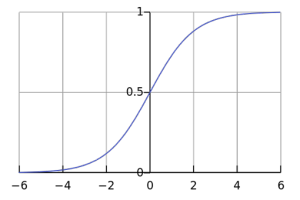Figure: The logistic sigmoid function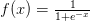. Image Source

A logistic regression model estimates the probability of a dependent variable as a function of independent variables. The dependent variable is the output (label) that we are trying to predict, while the independent variables or features are the factors that we feel could influence the output.

A generalized linear regression doesn’t need the data input to have a normal distribution. The test data can have any distribution. Logistic regression is a special case of the generalized linear regression where the response variable follows the logit function. The input of the logit function is a probability p, between 0 and 1. The odds ratio for probability p is defined as p/(1-p), and the logit function is defined as the logarithm of the Odds ratio or log-odds.

Logit(p) = Log(odds) = Log (p/(1-p))

The quality of a logistic regression model is determined by measures of fit and predictive power. R-squared is a measure of how well the independent variable in the logistic function can be predicted from the dependent variables, and ranges from 0 to 1. Many different ways exist to calculate R-square including the Cox-Snell R2 and the McFadden R2. Goodness of fit, on the other hand, can be measured using tests such as the Pearson chi-square, Hosmer-Lemeshow, and Stukel tests. The right type of test to use will depend on factors such as the distribution of p-values, interactions and quadratic effects, and grouping of data.

## Applications of Logistic Regression

Logistic regression is similar to a non-linear perceptron or a neural network without hidden layers. Logistic regression is most valuable for areas where data are scarce, like the medical and social sciences where it’s used to analyze and interpret results from experiments. Because regression is simple and fast, it’s also used for very large data sets. Logistic regression, however, cannot be applied to predict continuous outcomes or used with data sets that are not independent. There’s also a possibility of overfitting the model to the data when using logistic regression.

## Why Regression?

One of the principal uses of machine learning models is to forecast outcomes. This is also a primary value of regression analysis. Regression is a supervised learning technique that helps in finding the correlation between variables and enables the prediction of continuous outputs based upon one or more predictor variables. Machine learning algorithms can use different kinds of regression analysis for such functions as predicting the likelihood that a customer will buy a product based upon past purchase patterns, classifying objects by their characteristics and determining the impact that a change in a truck route will have on delivery scheduled.

Calculating the slope of the regression line using the least squares model is a time-consuming task that lends itself well to machine learning. The quality of outcomes and regression model also improves with the number of variables considered. Machine learning algorithms can factor in a much larger number of variables that humans, resulting in better outcomes as additional variables are introduced.

## Why Regression Runs Better on GPUs

Architecturally, the CPU is composed of just a few cores with lots of cache memory that can handle a few software threads at a time. In contrast, a GPU is composed of hundreds of cores that can handle thousands of threads simultaneously.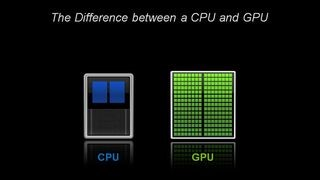Linear regression models use matrix multiplications that execute faster on GPUs. GPUs are also useful in calculating the loss function for linear regression, which maps decisions to their associated costs and is an essential element of least squares calculations. This requires computing a linear combination of elements and squaring them repeatedly, a task at which GPUs excel at.

## Logistic Regression in Deep Learning

In deep learning, the last layer of a neural network used for classification can often be interpreted as a logistic regression. In this context, one can see a deep learning algorithm as multiple feature learning stages, which then pass their features into a logistic regression that classifies an input.

Because neural nets are created from large numbers of identical neurons, they’re highly parallel by nature. This parallelism maps naturally to GPUs, providing a significant computation speed-up over CPU-only training. GPUs have become the platform of choice for training large, complex neural network-based systems for this reason, and the parallel nature of inference operations also lend themselves well for execution on GPUs.

The NVIDIA Deep Learning SDK provides powerful tools and libraries for designing and deploying GPU-accelerated deep learning applications including logistic regression. The Deep Learning SDK requires the NVIDIA® CUDA® Toolkit, which offers a comprehensive development environment for building GPU-accelerated deep learning algorithms.

## GPU-Accelerated, End-to-End Data Science

The NVIDIA RAPIDS suite of open-source software libraries, is built on CUDA primitives for low-level compute optimization, but exposes that GPU parallelism and high-bandwidth memory speed through user-friendly Python familiar interfaces.  RAPIDS gives the ability to execute end-to-end data science and analytics pipelines entirely on GPUs, while still using familiar interfaces like Pandas and Scikit-Learn APIs.

RAPIDS’s cuML machine learning algorithms and mathematical primitives follow the familiar scikit-learn-like API. Popular algorithms like Linear Regression, Logistic Regression, XGBoost, and many others are supported for both single GPU and large data center deployments. For large datasets, these GPU-based implementations can complete 10-50X faster than their CPU equivalents.With the RAPIDS GPU DataFrame, data can be loaded onto GPUs using a Pandas-like interface, and then used for various connected machine learning and graph analytics algorithms without ever leaving the GPU. This level of interoperability is made possible through libraries like Apache Arrow, which allows acceleration for end-to-end pipelines—from data prep to machine learning to deep learning.

RAPIDS supports device memory sharing between many popular data science libraries. This keeps data on the GPU and avoids costly copying back and forth to host memory.